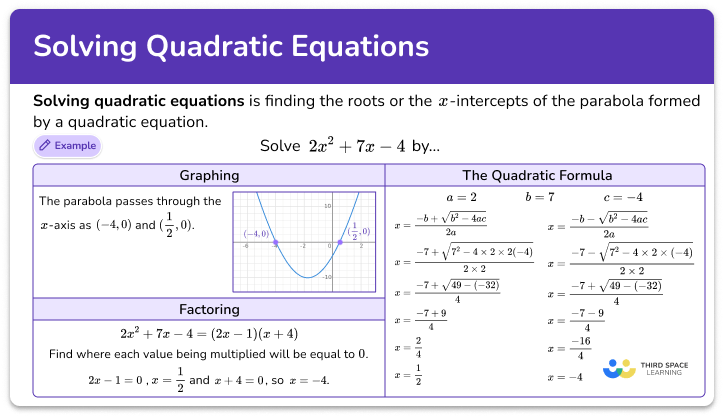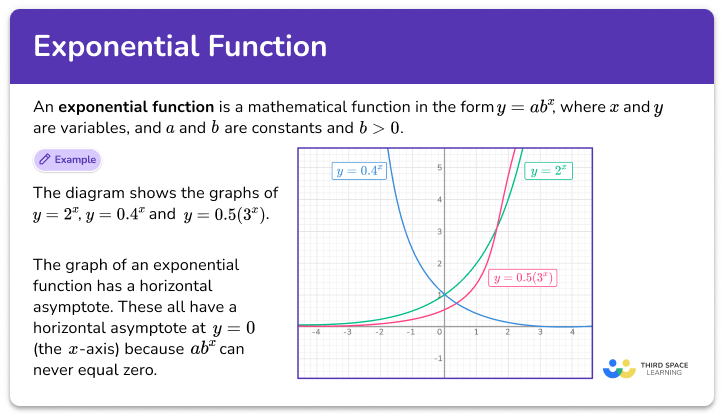Math resources Algebra

# Algebra

Here you will find all the algebra resources you need. Like all our math topic guides, we've included step-by-step guides, detailed examples and practice questions!

Filter by:

Algebraic expressions

Math equations

Types of graphs

Number patterns

Inequalities

Show all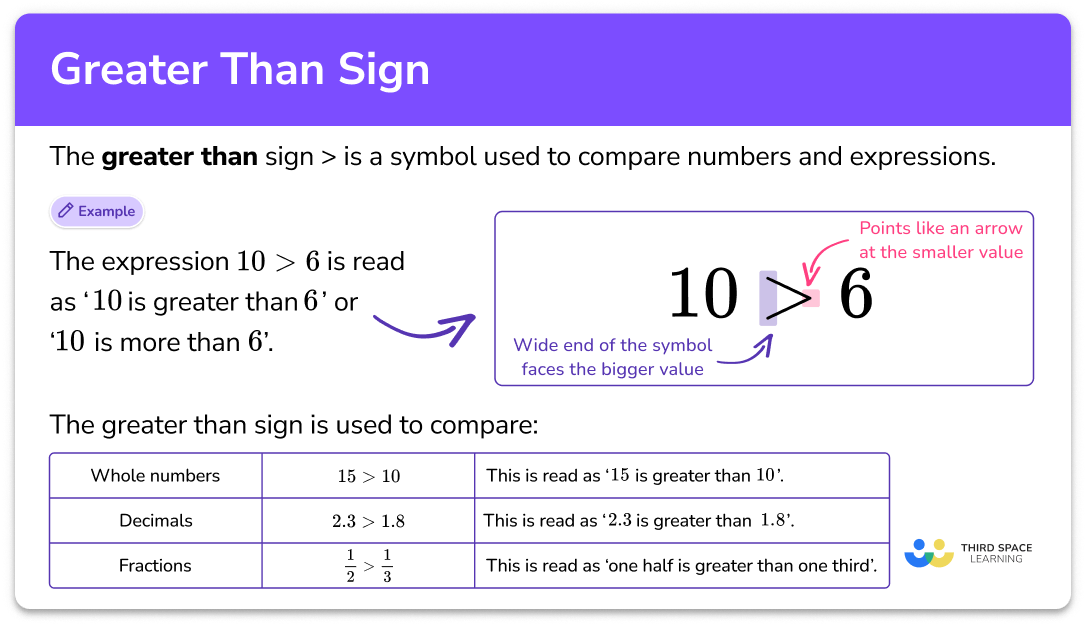Greater than sign

Greater than sign step-by-step topic guide, detailed examples, practice questions, teaching tips and FAQs.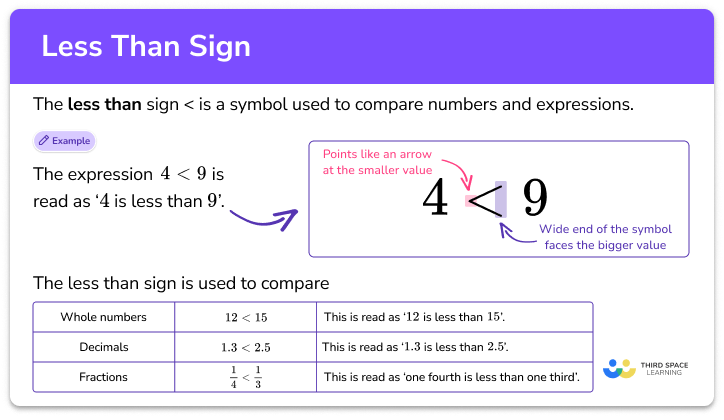Less than sign

Less than sign step-by-step topic guide, detailed examples, practice questions, teaching tips and FAQs.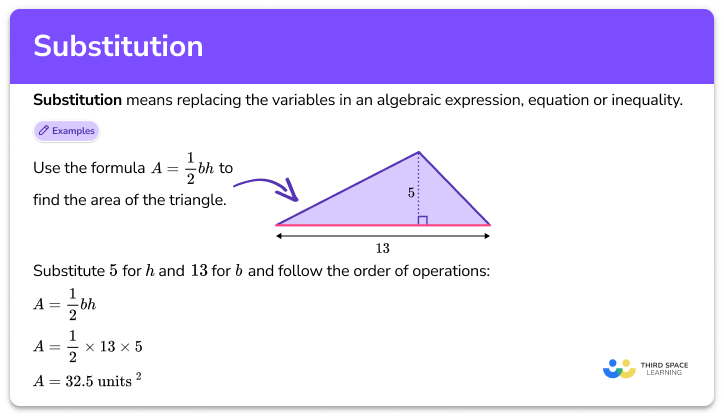Substitution

Substitution step-by-step topic guide, detailed examples, practice questions, teaching tips and FAQs.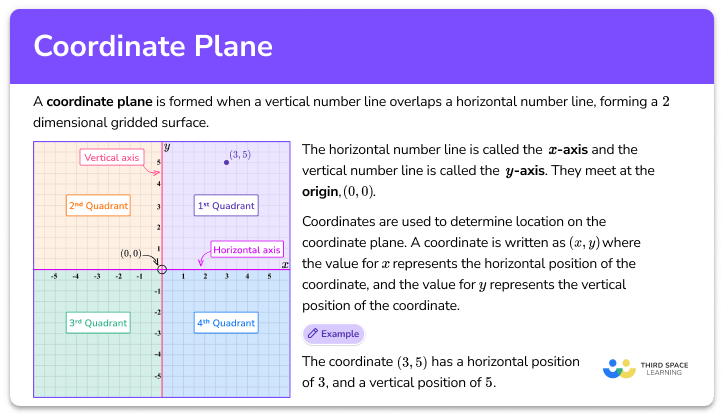Coordinate plane

Coordinate plane step-by-step topic guide, detailed examples, practice questions, teaching tips and FAQs.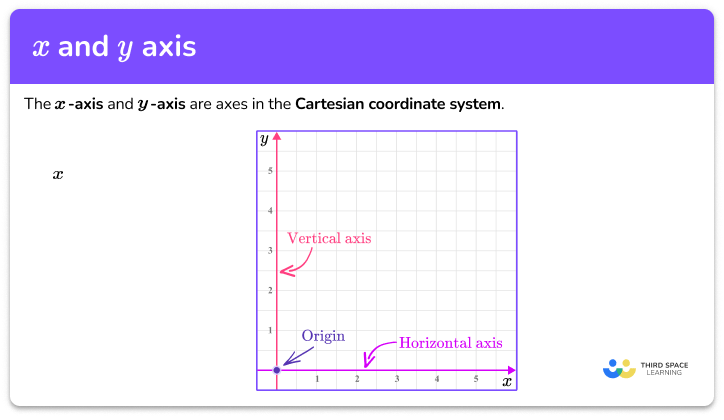x and y axis

x and y axis step-by-step topic guide, detailed examples, practice questions, teaching tips and FAQs.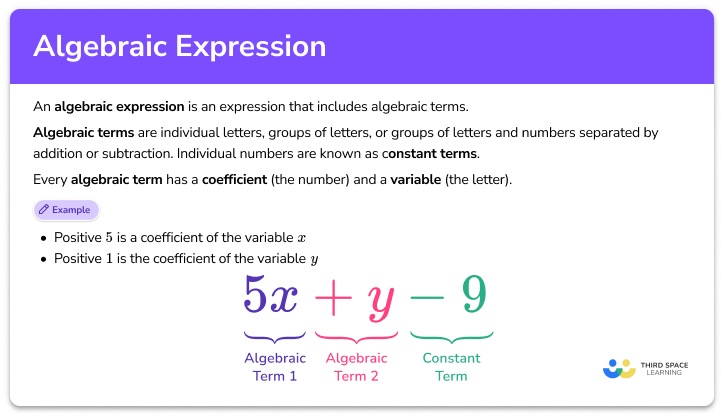Algebraic expression

Algebraic expression step-by-step topic guide, detailed examples, practice questions, teaching tips and FAQs.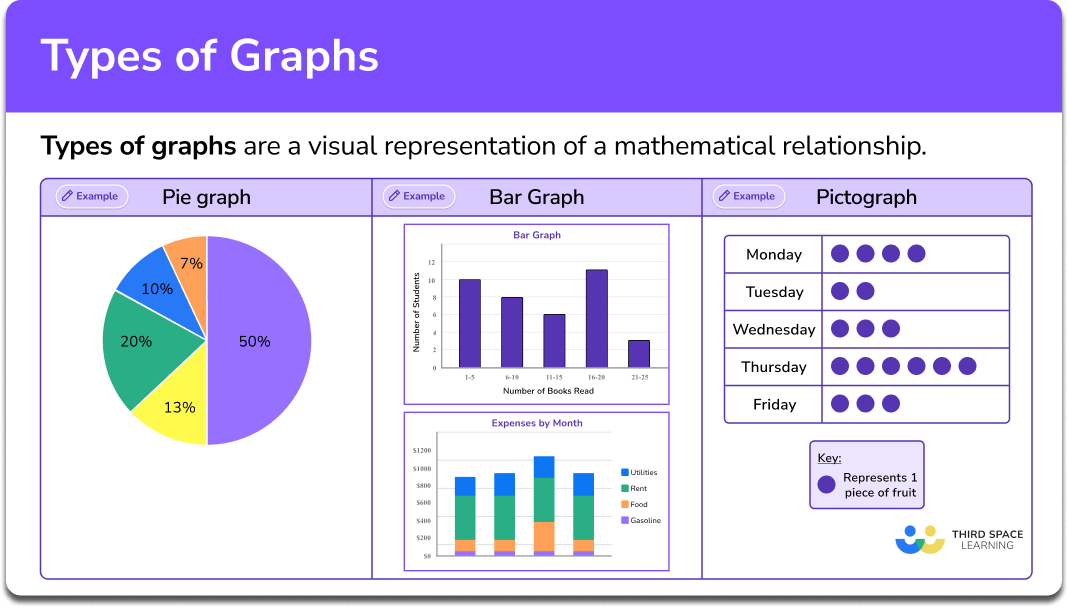Types of graphs

Types of graphs step-by-step topic guide, detailed examples, practice questions, teaching tips and FAQs.Linear graph

Linear graph step-by-step topic guide, detailed examples, practice questions, teaching tips and FAQs.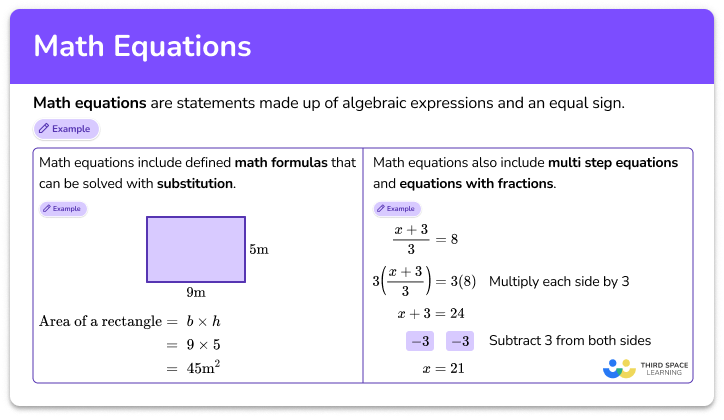Math equations

Math equations step-by-step topic guide, detailed examples, practice questions, teaching tips and FAQs.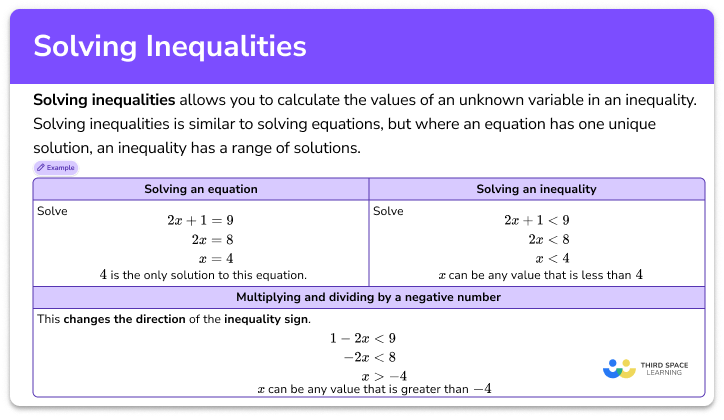Solving inequalities

Solving inequalities step-by-step topic guide, detailed examples, practice questions, teaching tips and FAQs.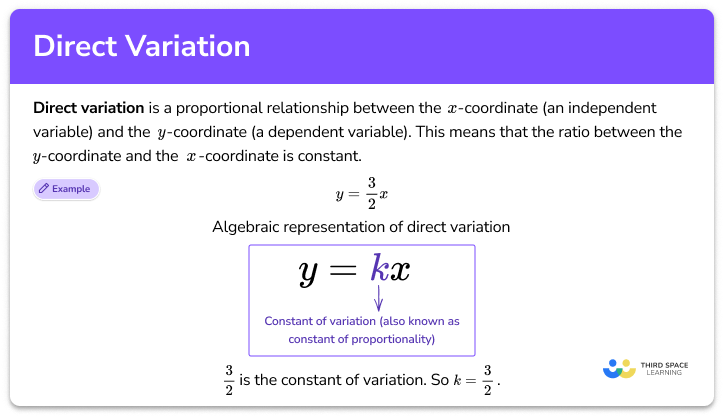Direct variation

Direct variation step-by-step topic guide, detailed examples, practice questions, teaching tips and FAQs.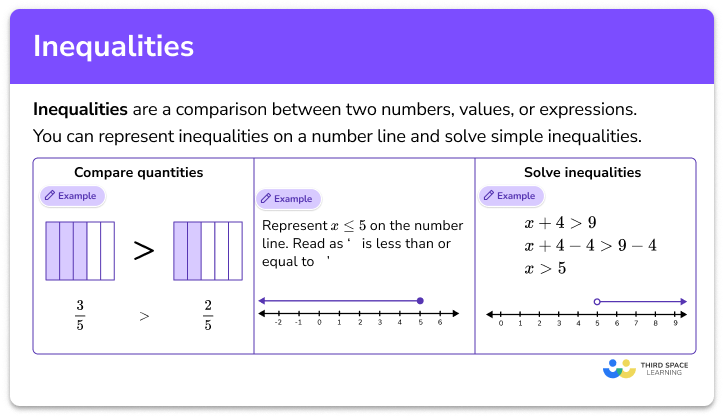Inequalities

Inequalities step-by-step topic guide, detailed examples, practice questions, teaching tips and FAQs.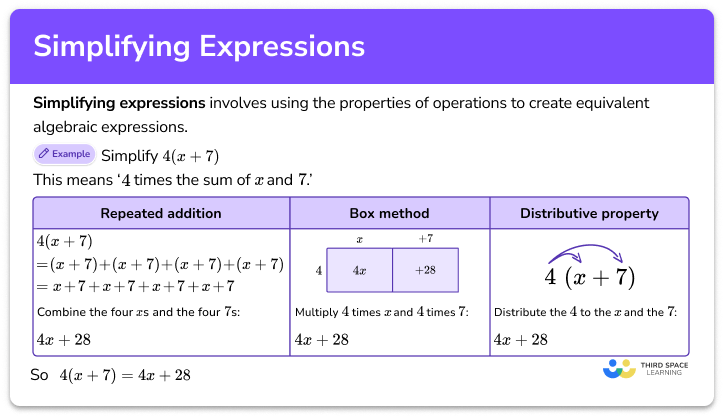Simplifying expressions

Simplifying expressions step-by-step topic guide, detailed examples, practice questions, teaching tips and FAQs.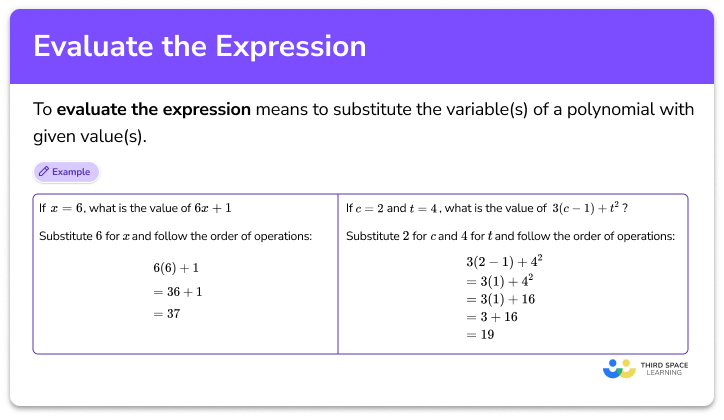Evaluate the expression

Evaluate the expression step-by-step topic guide, detailed examples, practice questions, teaching tips and FAQs.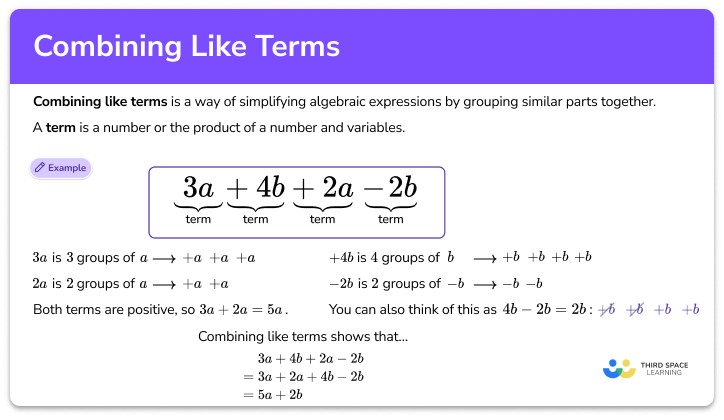Combining like terms

Combining like terms step-by-step topic guide, detailed examples, practice questions, teaching tips and FAQs.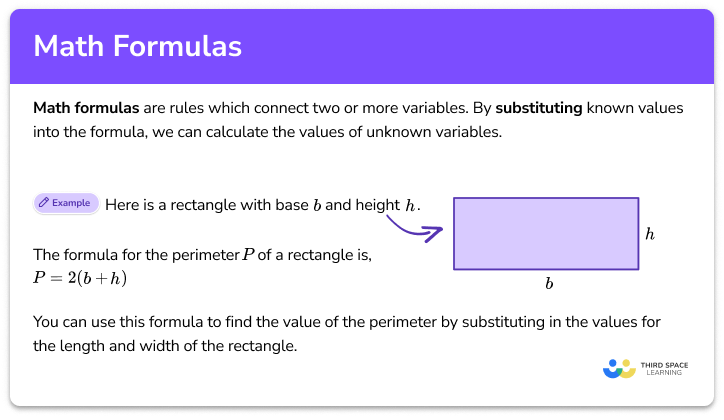Math formulas

Math formulas step-by-step topic guide, detailed examples, practice questions, teaching tips and FAQs.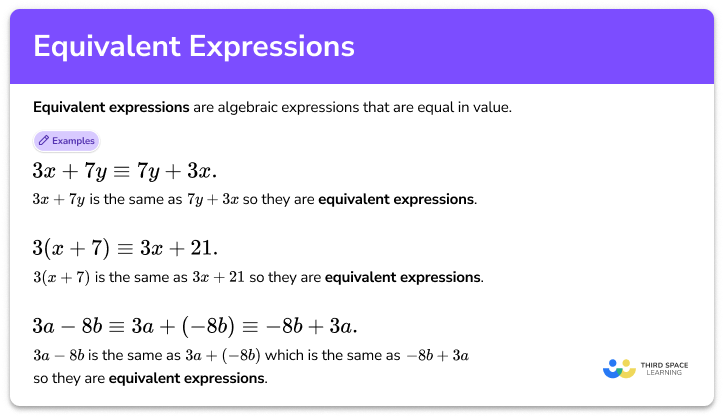Equivalent expressions

Equivalent expressions step-by-step topic guide, detailed examples, practice questions, teaching tips and FAQs.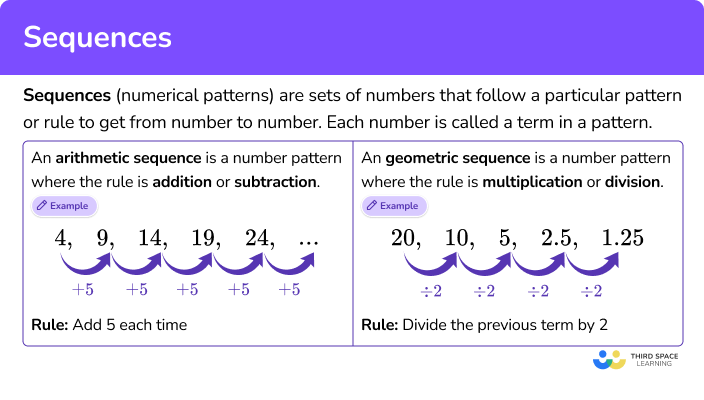Sequences

Sequences step-by-step topic guide, detailed examples, practice questions, teaching tips and FAQs.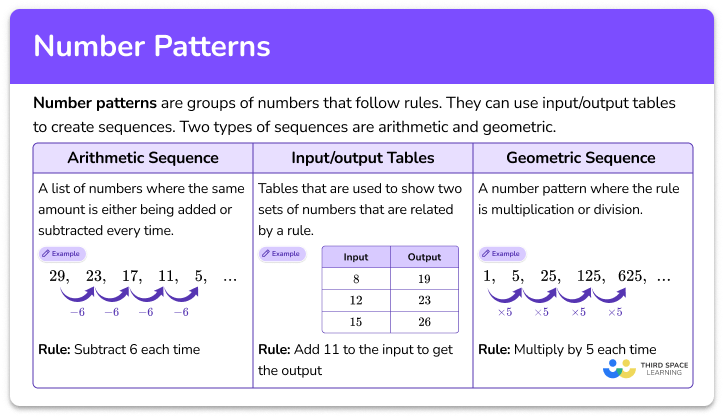Number patterns

Number patterns step-by-step topic guide, detailed examples, practice questions, teaching tips and FAQs.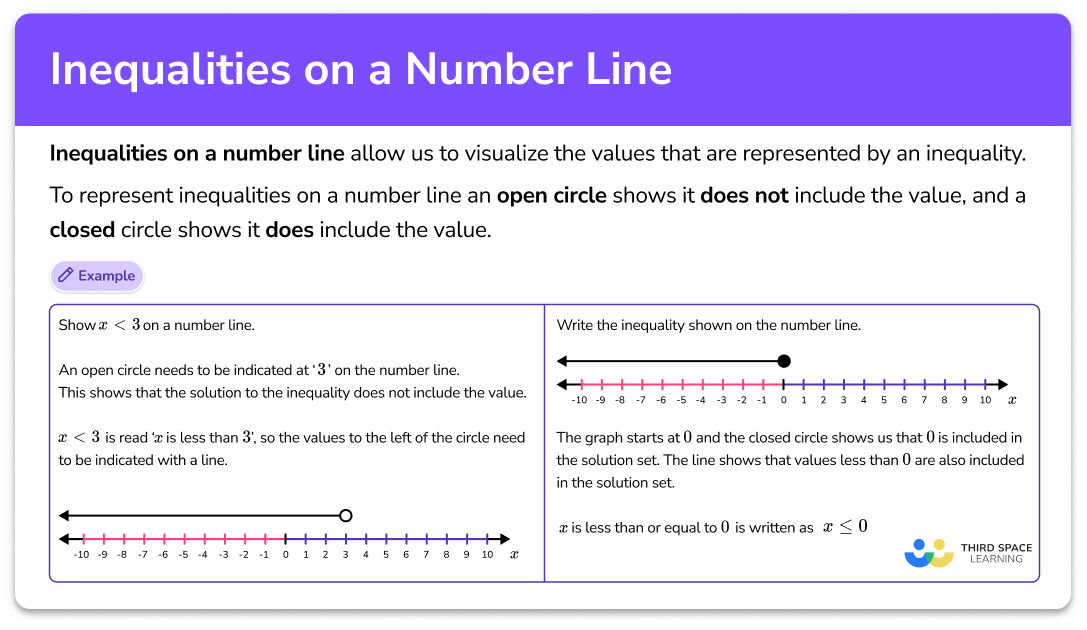Inequalities on a number line

Inequalities on a number line step-by-step topic guide, detailed examples, practice questions, teaching tips and FAQs.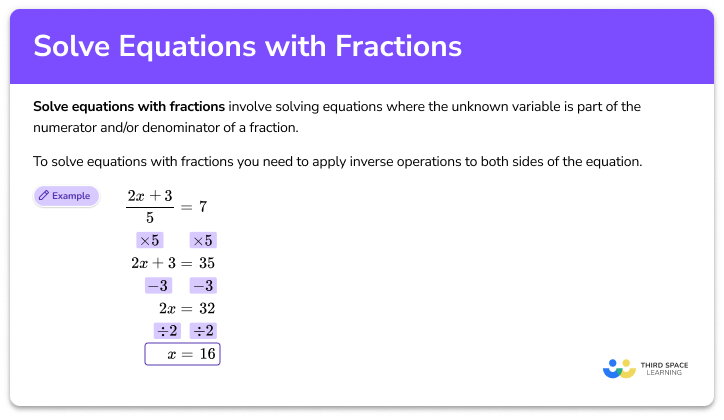Solve equations with fractions

Solve equations with fractions step-by-step topic guide, detailed examples, practice questions, teaching tips and FAQs.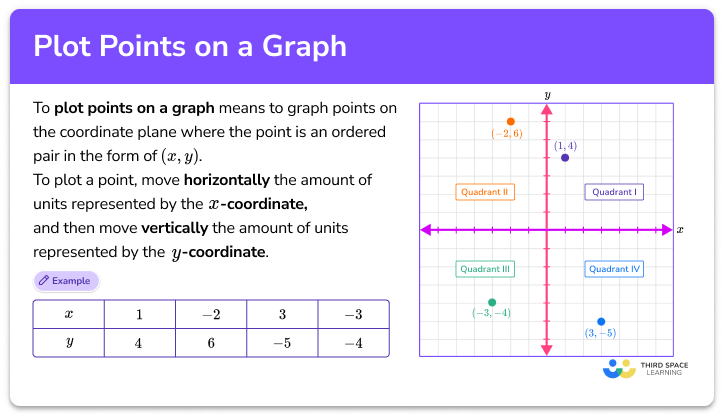Plot points on a graph

Plot points on a graph step-by-step topic guide, detailed examples, practice questions, teaching tips and FAQs.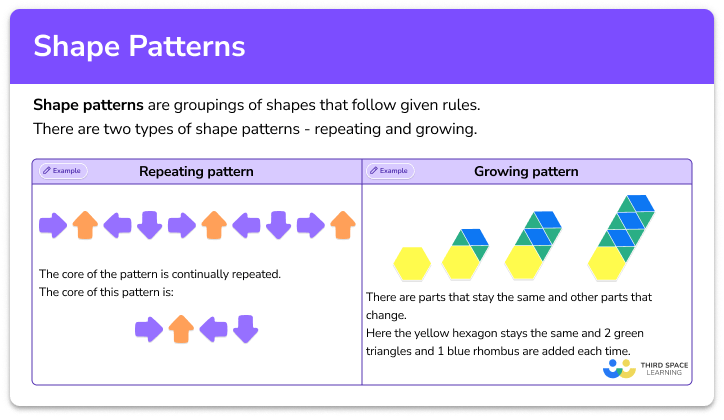Shape patterns

Shape patterns step-by-step topic guide, detailed examples, practice questions, teaching tips and FAQs.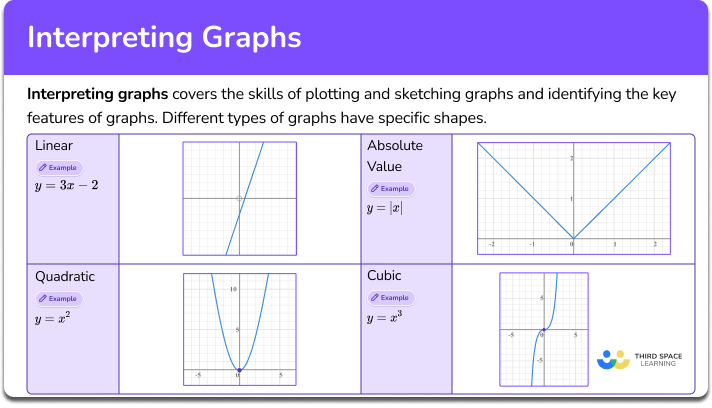Interpreting graphs

Interpreting graphs step-by-step topic guide, detailed examples, practice questions, teaching tips and FAQs.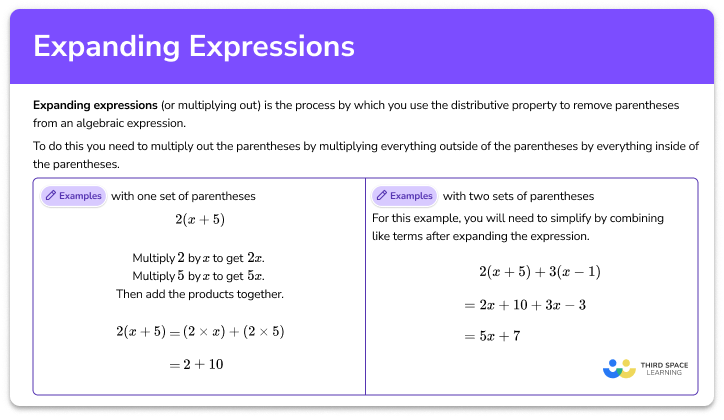Expanding expressions

Expanding expressions step-by-step topic guide, detailed examples, practice questions, teaching tips and FAQs.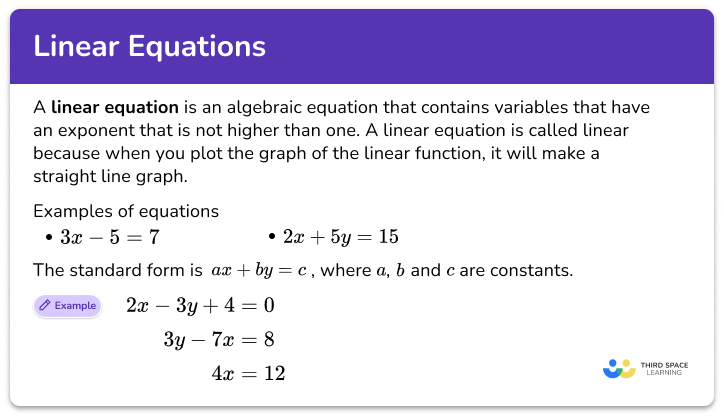Linear equations

Linear equations step-by-step topic guide, detailed examples, practice questions, teaching tips and FAQs.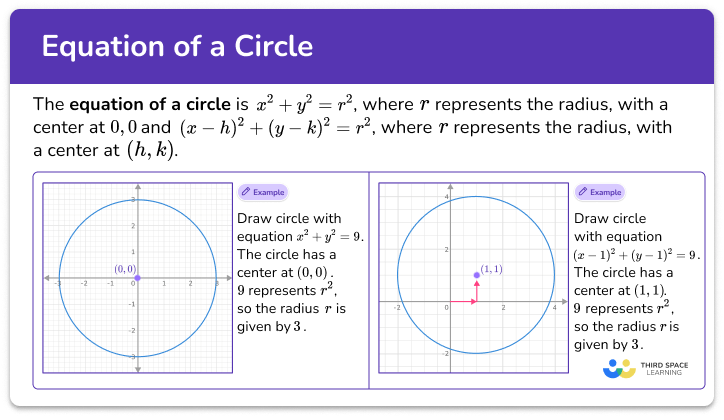Equation of a circle

Equation of a circle step-by-step topic guide, detailed examples, practice questions, teaching tips and FAQs.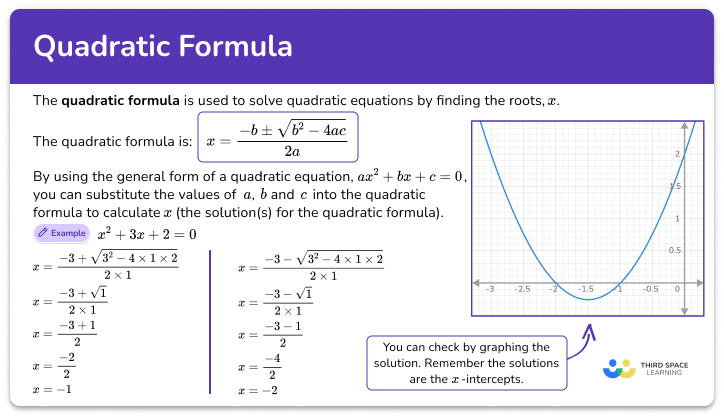Quadratic formula step-by-step topic guide, detailed examples, practice questions, teaching tips and FAQs.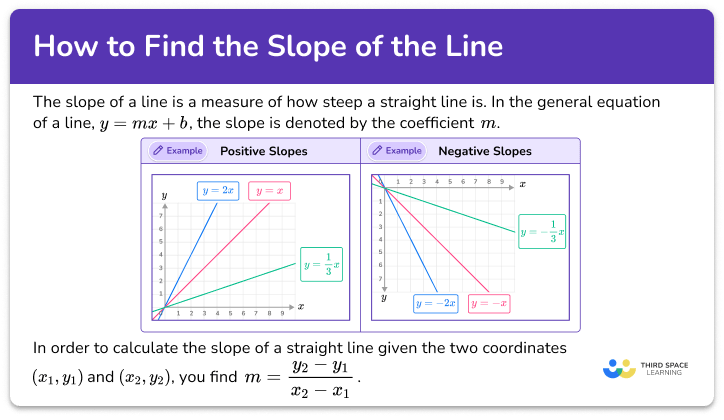How to find the slope of a line

How to find the slope of a line step-by-step topic guide, detailed examples, practice questions, teaching tips and FAQs.